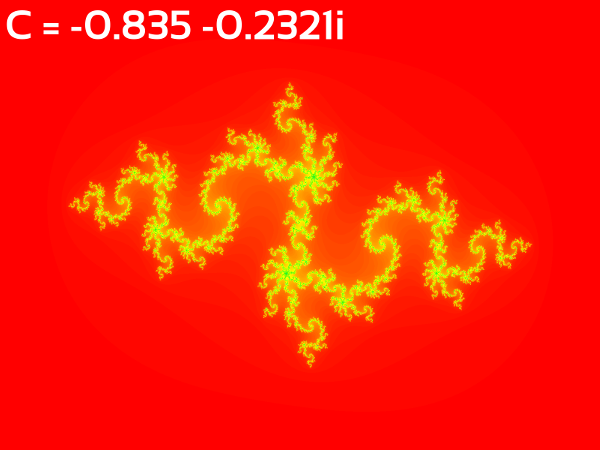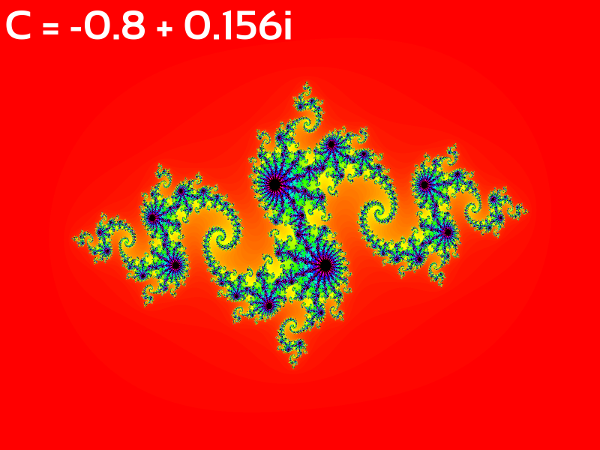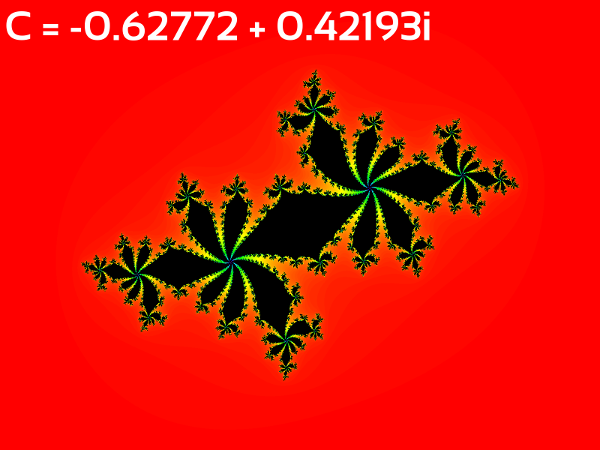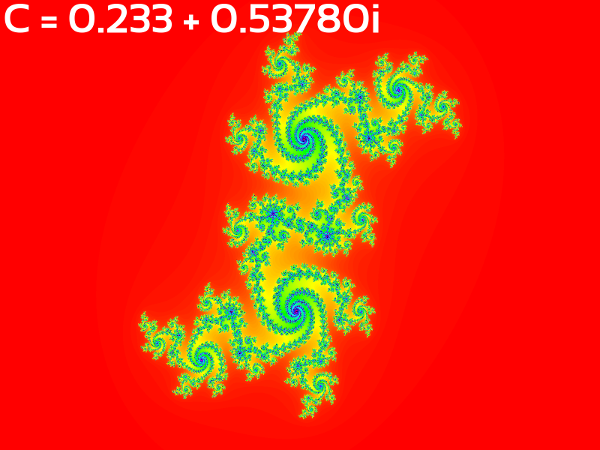### Fractal Advanced : Julia Set Basics (with Java Code)

Complex Numbers

Complex numbers are one of the most fascinating topics of mathematics. It'll be correct to say that this is a work of pure imagination because the basis of complex numbers are imaginary numbers. Any number (positive or negative) squared is always a positive entity so we cannot take the square root of a negative quantity? Well, apparently you can. Welcome to the world of complex numbers.

A complex number is reported in the form a + ib where i2=-1 hence i (read: iota) is the square root of -1 which isn't actually possible hence the coefficient multiplied with i i.e. b is called the imaginary part of complex number. Since an imaginary number cannot be represented on a real number line we cannot compare them i.e. we cannot say if sqrt(-6) is greater or sqrt(-2). The other coefficient a is called the real part of a complex number and can be represented on a real number line.

A complex number is generally represented by z where z = a + ib. The real part of the complex number is represented as Re(z) and the imaginary part as Im(z) where Re(z) = a and Im(z) = b.

Complex Arithmetic

i) (a + ib) + (c + id) = (a+c) + (b+d)i
ii) (a + ib)(c + id) = (ac-bd) + (ad+bc)i (since i2bd = -bd)

Modulus of a Complex Number

The modulus, magnitude or absolute value of a complex number is the square root of the sum of squares of its real (a) and imaginary (b) parts i.e. |z| = sqrt(a2 + b2).

Conjugate of a Complex Number

The conjugate of a complex number(z) is another complex number (z) with negated imaginary part of z i.e. z = a - ib.The Complex Conjugate (Source: Wikipedia)

The Complex Plane

The complex plane is a coordinate system where we can represent complex numbers. The real part is represented on the x-axis and the complex part on the y-axis.
z = x + iyThe Complex Plane (Source: Wikipedia)

A Circle in Complex Plane

An equation involving complex numbers is called a complex equation and can represent various geometrical shapes on the complex plane. Any equation of form |z| = c represents a circle with radius equal to c units. Let us analyze the equation by converting it into 2D real plane.

|z| = c in complex plane is equivalent to sqrt(x2 + y2) = c in the real plane. Squaring both sides of the equation we get x2 + y2 = c2 which is indeed the equation of a circle in 2D plane with radius c.

The Prisoner and Escapee Sets

Let there be a complex function f(z) and we feed a complex number z0 into it to get a resultant complex number z1 which is fed again into f to get a z2 and so on. We get a series of complex numbers {z0, z1, z2, z3 ...} which is called the orbit of z0 under f

For an example, let there be a complex function: f(z) = z2 - 0.5, taking z0 as 0 + 0i we get:

z1 = f(0) = -0.5
z2 = f(-0.5) = -0.25
z3 = f(-0.25) = -0.4375
z4 = f(-0.4375) = -0.30859375
z5 = f(-0.30859375) = -0.4047698974609375 and so on.

We see that f(z) never goes very far away from 0 + 0i hence we say that the orbit of z0 = (0+0i) is bounded and hence z0 is a prisoner

In the same function taking z0 as 4 + 0i we get:

z1 = f(4) = 15.5
z2 = f(15.5) = 239.75
z3 = f(239.75) = 57479.5625
z4 = f(57479.5625) = 3303900104.69140625 and so on.

We see that f(z) goes very far away from 4 + 0i on each iteration and hence we say that the orbit of z0 = (4+0i) is unbounded and hence z0 is an escapee

The Julia Set

The Julia set is the boundary between the prisoner set and the escape set. Let us for example take the simplest form of Julia set function f(z) = z2 + c where c is a constant complex number.If the orbit of any complex z0 ever leaves the circle of radius 2 (i.e. exceeds the value 2) then it goes far beyond 2 on further calculations and is kept in the escapee set. If however after a fixed number of calculations (let 256) the orbit does not go beyond 2 we can safely assume that z0 is a prisoner and is kept in prisoner set. Using a computer we can visualize the prisoner and escapee sets with different color tones and we get strikingly marvellous results which are fractals i.e. they repeat themselves even on the smallest scales.

Visualizing the Julia Set (The Algorithm)

We first assume an arbitrary complex number which will be the constant complex number c in the function zn+1 = zn + c. Then for every pixel (x,y) in the image we take a z0 which depends on x and y co-ordinates of the pixel in some way i.e. z0 = f(x,y) the simplest being z0 = x/ImageWidth + (y/ImageHeight)i where (x,y) is the location of the pixel on the image. We define a maximum number of iterations after which we can safely assume that the pixel belongs to prisoner set. Then we feed the z0 of each pixel into the function zn+1 = zn + c till the orbit exceeds the radius of 2 or the number of iterations exceed the maximum iterations. If the iterations stop due to orbit exceeding 2 the pixel is an escapee while if maximum iterations are reached the pixel is a prisoner. The pixel is then mapped to a color palette to generate the image, the simplest mapping being the following:

Hue = f(number of iterations taken to escape the orbit of 2)
Saturation = Constant
Brightness = 0 or 1 (depending on prisoner or escapee respectively)

So, a prisoner is definitely painted black because the Brightness is set to 0 for prisoners irrespective of the Hue.The escapees however have maximum Brightness (1) and Hue being a function of number of iterations taken to exceed 2 they are painted in different hues depending on the function. This gives rise to beautiful fractal designs.

The Java Implementation

First we need an implementation of complex numbers in Java so that we can make our task a bit easier. Here is a very simple implementation of Complex Numbers in Java:

```package plane.complex;

/**
* <code>ComplexNumber</code> is a class which implements complex numbers in Java.
* It includes basic operations that can be performed on complex numbers such as,
* addition, subtraction, multiplication, conjugate, modulus and squaring.
*
* @author      Abdul Fatir
* @version		1.0
*
*/
public class ComplexNumber
{
/**
* The real, Re(z), part of the <code>ComplexNumber</code>.
*/
private double real;
/**
* The imaginary, Im(z), part of the <code>ComplexNumber</code>.
*/
private double imaginary;
/**
* Constructs a new <code>ComplexNumber</code> object with both real and imaginary parts 0 (z = 0 + 0i).
*/

public ComplexNumber()
{
real = 0.0;
imaginary = 0.0;
}

/**
* Constructs a new <code>ComplexNumber</code> object.
* @param real the real part, Re(z), of the complex number
* @param imaginary the imaginary part, Im(z), of the complex number
*/

public ComplexNumber(double real, double imaginary)
{
this.real = real;
this.imaginary = imaginary;
}

/**
* Adds another <code>ComplexNumber</code> to the current complex number.
* @param complex_number the complex number to be added to the current complex number
*/

{
this.real = this.real + complex_number.real;
this.imaginary = this.imaginary + complex_number.imaginary;
}

/**
* The complex conjugate of the current complex number.
* @return a <code>ComplexNumber</code> object which is the conjugate of the current complex number
*/

public ComplexNumber conjugate()
{
return new ComplexNumber(this.real,-this.imaginary);
}

/**
* The modulus, magnitude or the absolute value of current complex number.
* @return the magnitude or modulus of current complex number
*/

public double mod()
{
return Math.sqrt(Math.pow(this.real,2) + Math.pow(this.imaginary,2));
}

/**
* The square of the current complex number.
* @return a <code>ComplexNumber</code> object which is the square of the current complex number
*/

public ComplexNumber square()
{
double _real = this.real*this.real - this.imaginary*this.imaginary;
double _imaginary = 2*this.real*this.imaginary;
return new ComplexNumber(_real,_imaginary);
}

/**
* Multiplies another <code>ComplexNumber</code> to the current complex number.
* @param complex_number the complex number to be multiplied to the current complex number
*/

public void multiply(ComplexNumber complex_number)
{
double _real = this.real*complex_number.real - this.imaginary*complex_number.imaginary;
double _imaginary = this.real*complex_number.imaginary + this.imaginary*complex_number.real;

this.real = _real;
this.imaginary = _imaginary;
}

/**
* Prints the complex number in x + yi format
*/

public void print()
{
System.out.println(this.real+" + "+this.imaginary+"i");
}
}
```

Now that we have complex numbers in Java, we are ready to implement the Julia set in Java. Here's the JuliaFractal.java which is capable of creating Julia set images. The code is explained in comments:

```import plane.complex.ComplexNumber;
import java.io.IOException;
import java.io.File;
import javax.imageio.ImageIO;
import java.awt.Color;
import java.awt.image.BufferedImage;
import static java.lang.System.out;

/**
* <code>JuliaFractal</code> is an executable class which can create Julia set fractals for input constant ComplexNumber.
* @author      Abdul Fatir
* @version		1.0
*
*/

public class JuliaFractal
{
public static void main(String args[])throws IOException
{
// Taking the Image WIDTH and HEIGHT variables. Increasing or decreasing the value will affect computation time.
double WIDTH = 1600;
double HEIGHT = 1200;

// Setting the Saturation of every pixel to maximum
// This can be played with to get different results
float Saturation = 1f;

// Creating a new blank RGB Image to draw the fractal on
BufferedImage img = new BufferedImage((int)WIDTH, (int)HEIGHT,BufferedImage.TYPE_3BYTE_BGR);

// Getting the constant ComplexNumber as input from the user for use in the function f(z) = z + c
out.print("Re(c): ");
out.print("Im(c): ");

// Creating the constant complex number from input real and imaginary values
ComplexNumber constant = new ComplexNumber(cReal,cImag);

// Setting the maximum iterations to 256. This can be increased if you suspect an escapee set may be found beyond this value.
// Increasing or decreasing the value will affect computation time.

int max_iter = 256;

// Looping through every pixel of image
for(int X=0; X<WIDTH; X++)
{
for(int Y=0; Y<HEIGHT; Y++)
{
// Creating an empty complex number to hold the value of last z
ComplexNumber oldz = new ComplexNumber();

// Setting the value of z0 for every pixel
// z0 is a function of (x,y) i.e. the pixel co-ordinates.
// The function can be anything, but should depend on (x,y) in some way and should lie between [-1,1]
// I use the function,
// Re(z) = 2*(X-WIDTH/2)/(WIDTH/2)
// Im(z) = 1.33*(Y-HEIGHT/2)/(HEIGHT/2)
// This gives a good centered fractal.You can play around with the function to get better results.

ComplexNumber newz = new ComplexNumber(2.0*(X-WIDTH/2)/(WIDTH/2), 1.33*(Y-HEIGHT/2)/(HEIGHT/2) );

// Iterating till the orbit of z0 escapes the radius 2 or till maximum iterations are completed
int i;
for(i=0;i<max_iter; i++)
{
// Saving the current z in oldz
oldz = newz;

// Applying the function newz = newz^2 + c, where c is the constant ComplexNumber user input
newz = newz.square();

// Checking if the modulus/magnitude of complex number has exceeded the radius of 2
// If yes, the pixel is an escapee and we break the loop
if(newz.mod() > 2)
break;
}

// Checking if the pixel is an escapee
// If yes, setting the brightness to the maximum
// If no, setting the brightness to zero since the pixel is a prisoner
float Brightness = i < max_iter ? 1f : 0;

// Setting Hue to a function of number of iterations (i) taken to escape the radius 2
// Hue = (i%256)/255.0f;
// i%256 to bring i in range [0,255]
// Then dividing by 255.0f to bring it in range [0,1] so that we can pass it to Color.getHSBColor(H,S,B) function
float Hue = (i%256)/255.0f;

// Creating the color from HSB values and setting the pixel to the computed color
Color color = Color.getHSBColor(Hue, Saturation, Brightness);
img.setRGB(X,Y,color.getRGB());
}
}
// Saving the image
ImageIO.write(img,"PNG", new File("julia.png"));
}
}
```

Example Outputsc = -0.4 + 0.6ic = -0.673 + 0.0123ic = 0.285 + 0ic = 0.285 + 0.01ic = -0.70176 - 0.3842ic = -0.835 - 0.232ic = -0.8 + 0.156ic = -0.62772 + 0.42193ic = 0.233 + 0.53780ic = -0.7709787 - 0.08545i

We can definitely add better colors using different color mapping techniques and color smoothing algorithms to get a perfect gradient. This will be covered in an advanced article on Julia set. These are just the results of a two-degree complex function, Julia set however is not restricted to two-degree equations. There are cubic, bi-quadratic, exponential and loads of other complex functions which can power the Julia set. These will also be covered in the next article.